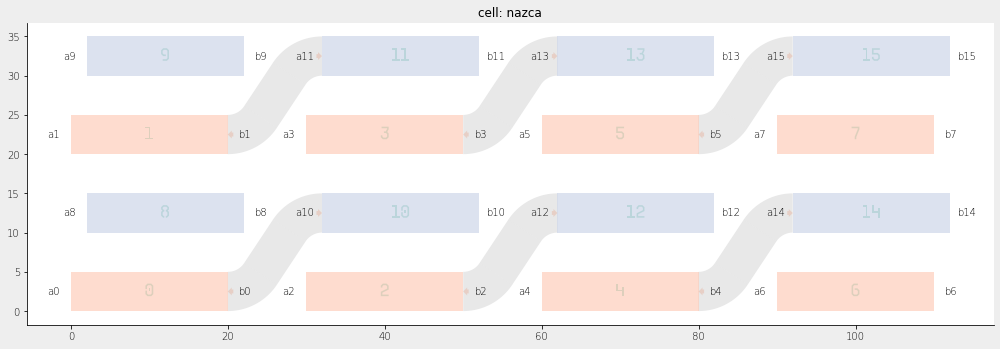Home Forums Nazca Connect polygons corresponding to different layers Reply To: Connect polygons corresponding to different layers

#6485

Hi A,

Below is an example to achieve what you want. Depending on your polygons etc. you may want to add a more fancy polygon analysis, but that is not Nazca specific.

``````import nazca as nd
import nazca.geometries as geom
from nazca.interconnects import Interconnect

def rect1(layer_n=1):
rect_shape = nd.geom.rectangle(length=20, height=5)
with nd.Cell('rect1') as cell:
nd.Polygon(layer=1, points=rect_shape).put(0)
nd.Polygon(layer=10, points=rect_shape).put(2, 10)
# guide=ic.sbend_p2p(pin1=r1, pin2=r2.pin['a0']).put()
return cell

rect1().put(array=[4, [30, 0], 2, [0, 20]])  # This could be a gds_load() based cell.

# 1. Extract all the polygon from cell into a dict with key (layer, x, y),
#    where x and y will be, just a choice, the geometrical average of the polygon points.
polygons = {}
for params in nd.cell_iter(nd.cfg.topcell, flat=True):
for pgon, points, bbox in params.iters['polygon']:
xp, yp = zip(*points)
xavg, yavg = round(sum(xp) / len(xp), 2), round(sum(yp) / len(yp), 2)
polygons[(pgon.layer, xavg, yavg)] = points

# 2. Analyse polygons: order them and extract positions for pins.
for i, ((layer, x, y), points) in enumerate(sorted(polygons.items())):
nd.text(str(i), height=2, align='cc', layer=0).put(x, y)
sort = sorted(points)
x0 = sort + 0.5 * (sort - sort)
y0 = sort + 0.5 * (sort - sort)
w0 = abs(sort - sort)
nd.Pin(f'a{i}', width=w0).put(x0, y0, 180)
x1 = sort[-1] + 0.5 * (sort[-2] - sort[-1])
y1 = sort[-1] + 0.5 * (sort[-2] - sort[-1])
nd.Pin(f'b{i}', width=w0).put(x1, y1, 0)

for i, (name, pin) in enumerate(nd.cfg.topcell.pin.items()):
if i in [0, 1, 2, 3, 4, 5]:
ic.bend_strt_bend_p2p(
pin1=nd.cfg.topcell.pin[f'b{i}'],
pin2=nd.cfg.topcell.pin[f'a{i+10}'],
).put()

nd.export_plt()``````

Note that it would make sense to put item 2 and 3 in the script above inside a new cell `with nd.Cell("newcellname") as C:`, and work with cell C, rather than working on the default topcell under nd.cfg.topcell.

Ronald